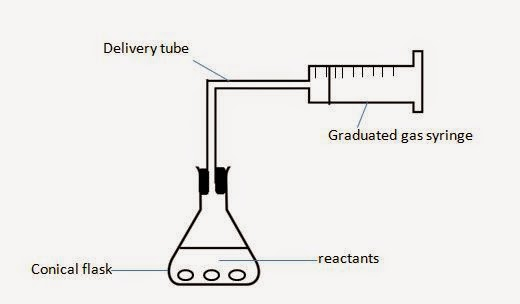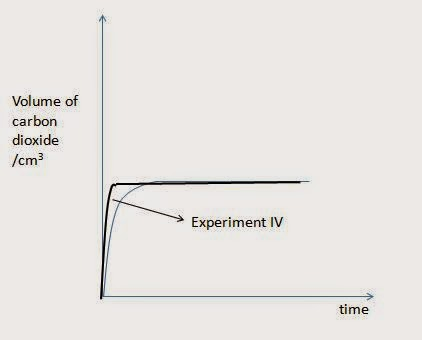Wednesday, 15 July 2015

Rate of reaction

1. Introduction
In order for reaction to take place, reacting particles need to collide in the desired orientation, with a certain minimum of energy This energy is known as activation energy.

Activation energy is the minimum energy required for the reaction to take place.

2. Factors affecting rate of reaction
• concentration of solution
When the concentration of the solution increases, the amount of particles per unit volume increases. Frequency of collision between reacting particles increases. Rate of reaction increases due to increase in frequency of effective collision.
• pressure of gases
When the pressure of gases increases, the amount of particles per unit volume increases. Frequency collision between reacting particles increases. Rate of reaction increases due to increase in frequency of effective collision.
• particle size of solids
When the size of solids decreases, surface area of the solid particles increases, allowing them to collide with other reacting particles more readily. Rate of reaction increases due to increase in frequency of effective collision.
• temperature
When temperature increases, average kinetic energy of the particles increases. Proportion of particles with energy greater than or equal to activation energy increases. Rate of reaction increases due to increase in frequency of effective collision.
• catalyst
Catalyst increases the rate of reaction by providing an alternative pathway, one with a lower activation energy. More particles will have energy greater than or equal to activation energy. Frequency of effective collision increases, and hence rate of reaction increases.

Note that the catalyst remains chemically unchanged at the end of the reaction.

3. Experimental setup to follow rate of reaction
If a reaction gives out gases, the following setup could be used to study the rate of reaction, by obtaining the volume of gas evolved at regular time intervals..

3.1 Measuring change in mass with time, to follow rate of reaction.Rate of reaction experiments - measuring change in mass with time

3.2 Measuring volume of gas produced with time, to follow rate of reaction.Rate of reaction experiments - measuring change in volume of gas with time

4. Interpret data obtained from experiments concerned with speed of reaction
Example. An experiment was set up to study the rate of reaction between excess powdered calcium carbonate and 20 cmof 0.25 mol/dm3 sulfuric acid at 35oC. The volume of carbon dioxide evolved with time is plotted on a graph below:Rate of reaction experiments -  graph of volume of gas evolved with time
i) Explain why does the gradient of the graph decreases until it reaches zero.
The gradient of the graph represents the rate of reaction.
As the reaction progresses, concentration of the acid decreases. Rate of reaction decreases. When all the acid (which is the limiting reagent) is used up, reaction stops, and gradient of graph is zero.

ii) A further experiment, experiment II was repeated, using calcium carbonate granules. Sketch a curve on the graph to show the pattern of results for this experiment. Label the graph II.
Granules are of larger size than powder. The larger the particles size, the smaller the surface area, and the lower the rate of reaction.
There is no change in the amount of calcium carbonate and sulfuric acid used. Hence, maximum volume of gas remains the same.Effect of surface area of solids on rate of reaction

iii) Another experiment, experiment III was repeated, using the same conditions as experiment I, except that 10 cmof 0.5 mol/dm3 of sulfuric acid is used. Sketch a curve on the graph to show the pattern of results for this experiment. Label the graph III.
Concentration of acid increases, hence rate of reaction increases. As sulfuric acid is the limiting reagent, any change in its amount will affect the volume of gas produced.
From the first experiment, the no. of moles of sulfuric acid = 20/1000 x 0.25 = 0.005 mol
For experiment III, the no. of moles of sulfuric acid = 10/1000 x 0.5 = 0.005 mol
Since no. of moles of limiting reagent (sulfuric acid) is the same, volume of gas produced is the same.Effect of concentration on rate of reaction

iv) In experiment IV, the experiment is conducted at 50 oC . Sketch a curve on the graph to show the pattern of results for this experiment. Label the graph IV.
Number of moles of reactant remains the same, only temperature has changed. Hence, no change in maximum volume of gas produced.
The higher the temperature, the higher the rate of reaction. Hence, graph IV will have a higher rate than the first experiment.Effect of temperature on rate of reaction,

Questions